### Home > A2C > Chapter 2 > Lesson 2.2.2 > Problem2-135

2-135.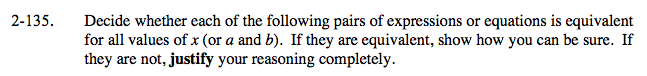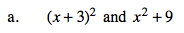Try substituting numbers for x and simplifying each expression. 0 and 1 can give different results, so check them as well as other numbers.

These two expressions are not equivalent. (But if you had only used 0 you might have thought they were).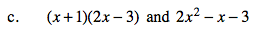Multiply and simplify.

These two expressions are equivalent.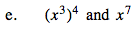See part (a).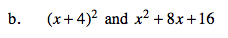Remember that (x + 4)2 means (x + 4)(x + 4) and multiply.

These two expressions are equivalent.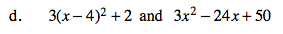Simplify the first expression and compare. Be sure you read the hint in part (b).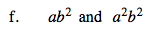Try substituting numbers for a and b.